# Software Engineering Software Cost Estimation Software Engineering SW

• Slides: 91Software Engineering Software Cost Estimation Software Engineering SW Cost Estimation Slide 1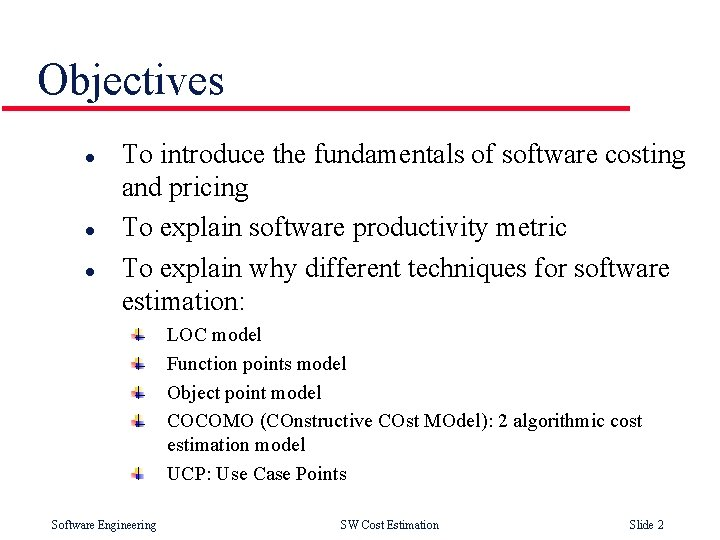Objectives l l l To introduce the fundamentals of software costing and pricing To explain software productivity metric To explain why different techniques for software estimation: LOC model Function points model Object point model COCOMO (COnstructive COst MOdel): 2 algorithmic cost estimation model UCP: Use Case Points Software Engineering SW Cost Estimation Slide 2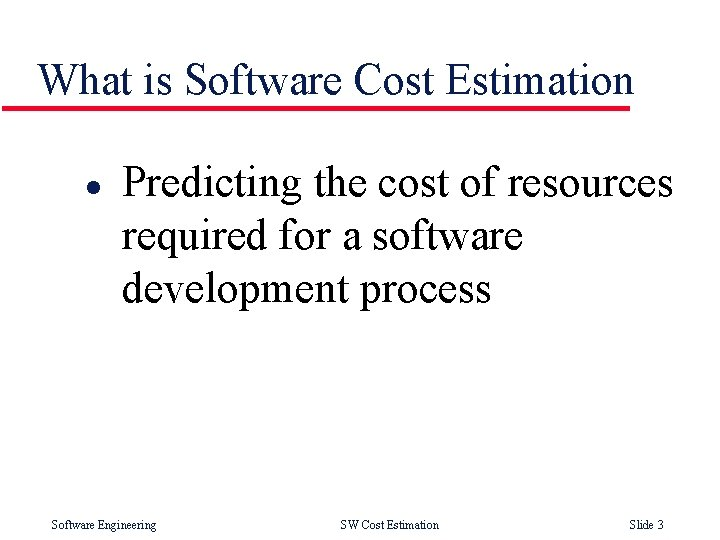What is Software Cost Estimation l Predicting the cost of resources required for a software development process Software Engineering SW Cost Estimation Slide 3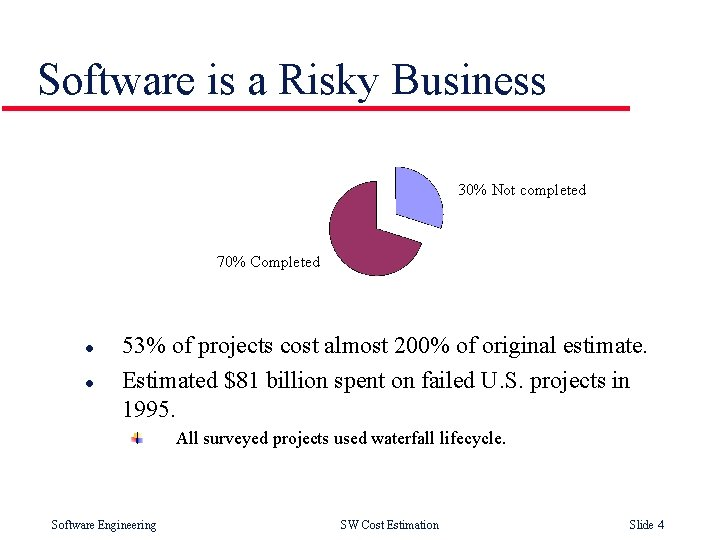Software is a Risky Business 30% Not completed 70% Completed l l 53% of projects cost almost 200% of original estimate. Estimated \$81 billion spent on failed U. S. projects in 1995. All surveyed projects used waterfall lifecycle. Software Engineering SW Cost Estimation Slide 4Software is a Risky Business l British Computer Society (BCS) survey: 1027 projects Only 130 were successful ! l Success was defined as: deliver all system requirements within budget within time to the quality agreed on Software Engineering SW Cost Estimation Slide 5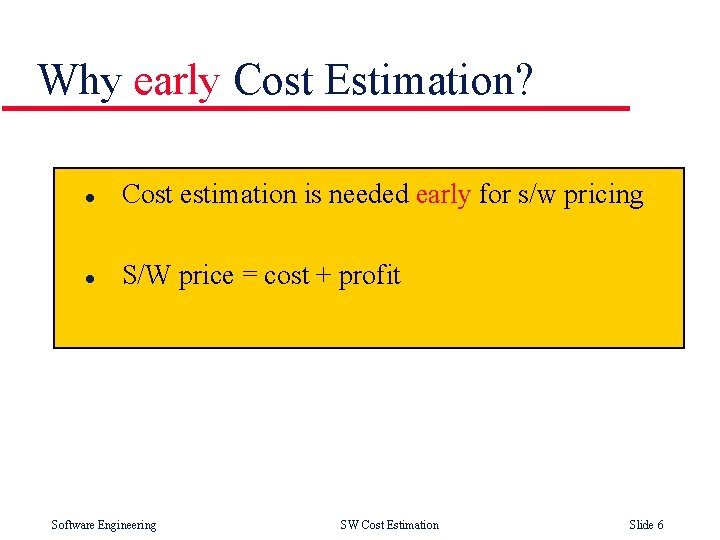Why early Cost Estimation? l Cost estimation is needed early for s/w pricing l S/W price = cost + profit Software Engineering SW Cost Estimation Slide 6Fundamental estimation questions l Effort How much effort is required to complete an activity? Units: man-day (person-day), man-week, man-month, , . . l Duration How much calendar time is needed to complete an activity? Resources assigned Units: hour, day, week, month, year, . . l Cost of an activity What is the total cost of an activity? l Project estimation and scheduling are interleaved management activities Software Engineering SW Cost Estimation Slide 7Software Cost Components 1. Effort costs (dominant factor in most projects) salaries Social and insurance & benefits 2. Tools costs: Hardware and software for development Depreciation on relatively small # of years 300 K US\$ 3. Travel and Training costs (for particular client) 4. Overheads(OH): Costs must take overheads into account costs of building, air-conditioning, heating, lighting costs of networking and communications (tel, fax, ) costs of shared facilities (e. g library, staff restaurant, etc. ) depreciation costs of assets Activity Based Costing (ABC) Software Engineering SW Cost Estimation Slide 8S/W Pricing Policy S/W price is influenced by l economic consideration l political consideration l and business consideration Software Engineering SW Cost Estimation Slide 9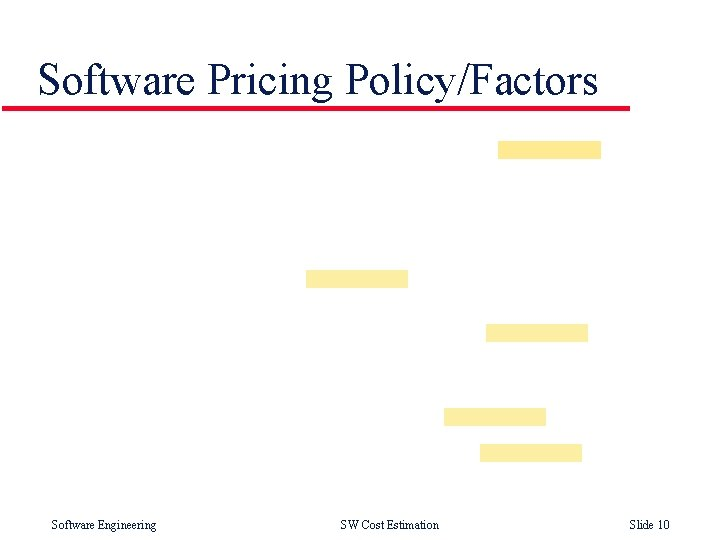Software Pricing Policy/Factors Software Engineering SW Cost Estimation Slide 10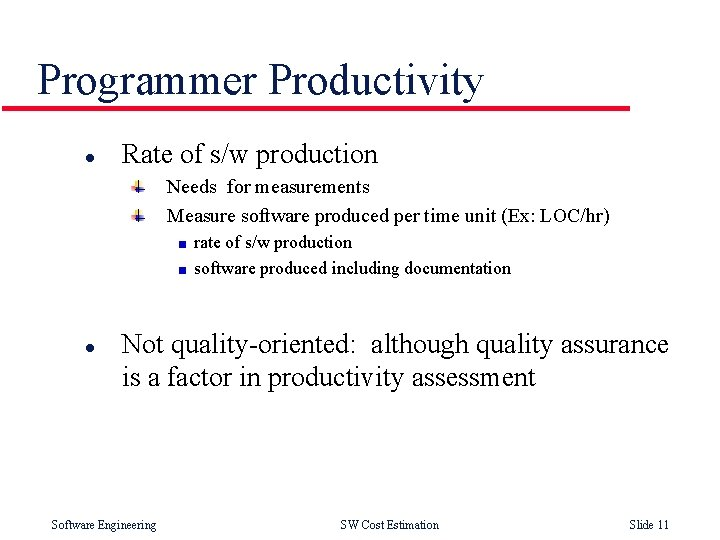Programmer Productivity l Rate of s/w production Needs for measurements Measure software produced per time unit (Ex: LOC/hr) rate of s/w production software produced including documentation l Not quality-oriented: although quality assurance is a factor in productivity assessment Software Engineering SW Cost Estimation Slide 11Productivity measures S/W productivity measures are based on: l Size related measures: Based on some output from the software process Number lines of delivered source code (LOC) l Function-related measures based on an estimate of the functionality of the delivered software: Function-points (are the best known of this type of measure) Object-points UCP Software Engineering SW Cost Estimation Slide 12Measurement problems l l l Estimating the size of the measure Estimating the total number of programmermonths which have elapsed Estimating contractor productivity (e. g. documentation team) and incorporating this estimate in overall estimate Software Engineering SW Cost Estimation Slide 13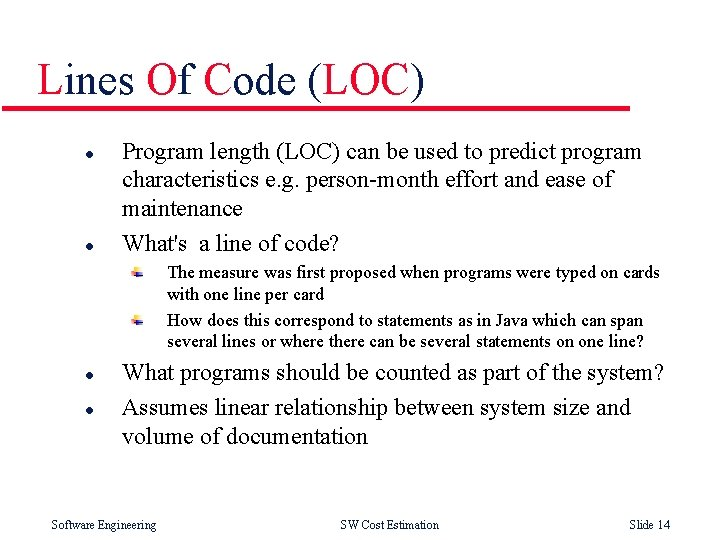Lines Of Code (LOC) l l Program length (LOC) can be used to predict program characteristics e. g. person-month effort and ease of maintenance What's a line of code? The measure was first proposed when programs were typed on cards with one line per card How does this correspond to statements as in Java which can span several lines or where there can be several statements on one line? l l What programs should be counted as part of the system? Assumes linear relationship between system size and volume of documentation Software Engineering SW Cost Estimation Slide 14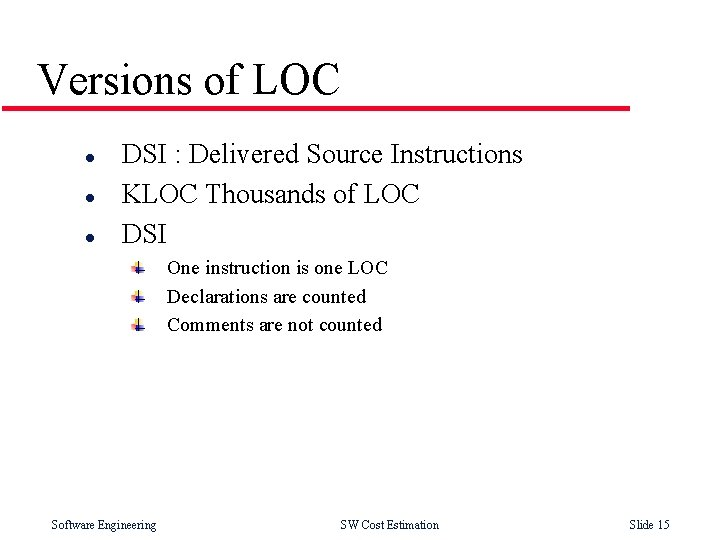Versions of LOC l l l DSI : Delivered Source Instructions KLOC Thousands of LOC DSI One instruction is one LOC Declarations are counted Comments are not counted Software Engineering SW Cost Estimation Slide 15LOC l Advantages Simple to measure l Disadvantages Defined on code: it can not measure the size of specification Based on one specific view of size: length. . What about complexity and functionality !! Bad s/w may yield more LOC Language dependent l Therefore: Other s/w size attributes must be included Software Engineering SW Cost Estimation Slide 16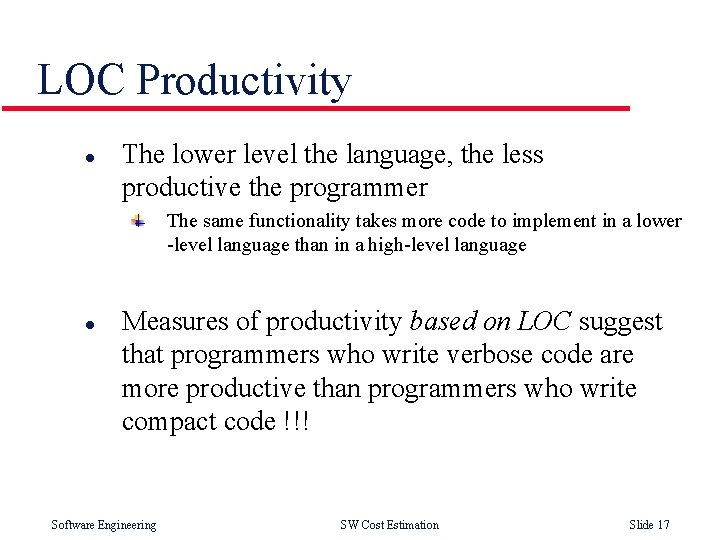LOC Productivity l The lower level the language, the less productive the programmer The same functionality takes more code to implement in a lower -level language than in a high-level language l Measures of productivity based on LOC suggest that programmers who write verbose code are more productive than programmers who write compact code !!! Software Engineering SW Cost Estimation Slide 17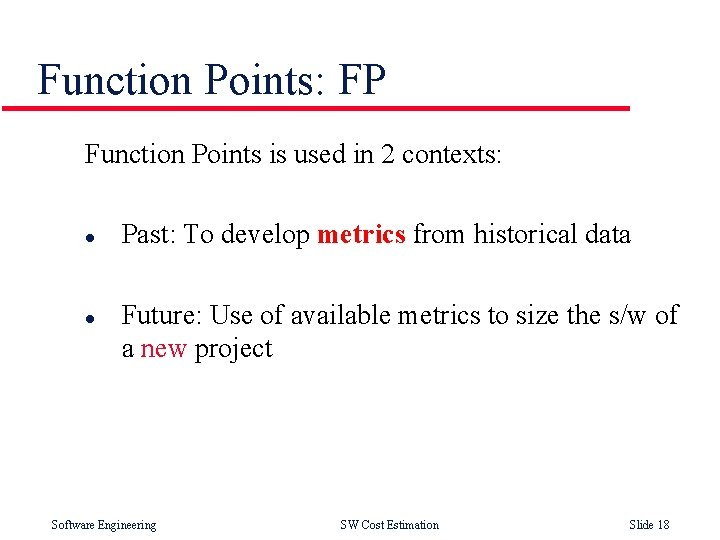Function Points: FP Function Points is used in 2 contexts: l l Past: To develop metrics from historical data Future: Use of available metrics to size the s/w of a new project Software Engineering SW Cost Estimation Slide 18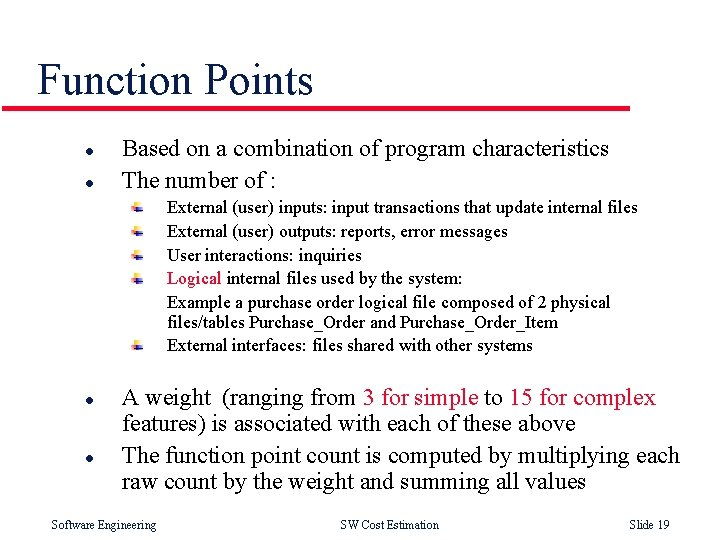Function Points l l Based on a combination of program characteristics The number of : External (user) inputs: input transactions that update internal files External (user) outputs: reports, error messages User interactions: inquiries Logical internal files used by the system: Example a purchase order logical file composed of 2 physical files/tables Purchase_Order and Purchase_Order_Item External interfaces: files shared with other systems l l A weight (ranging from 3 for simple to 15 for complex features) is associated with each of these above The function point count is computed by multiplying each raw count by the weight and summing all values Software Engineering SW Cost Estimation Slide 19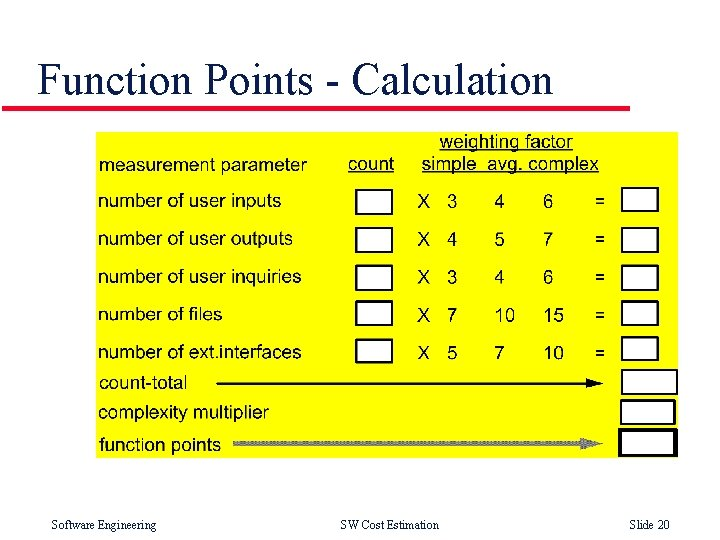Function Points - Calculation Software Engineering SW Cost Estimation Slide 20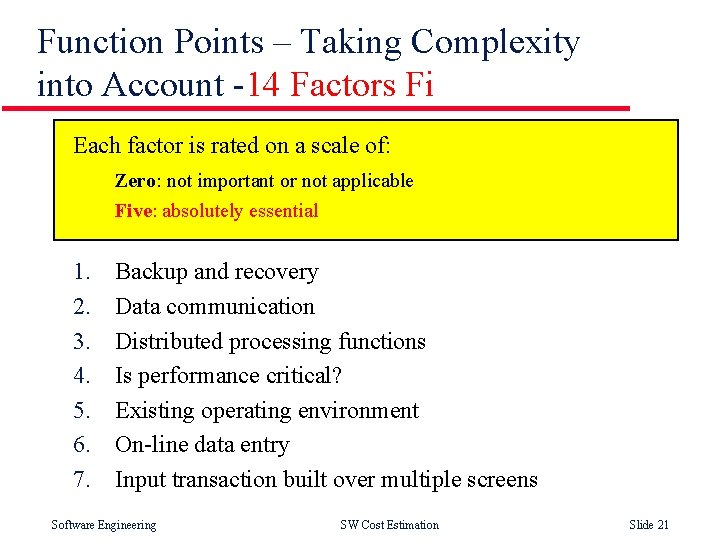Function Points – Taking Complexity into Account -14 Factors Fi Each factor is rated on a scale of: Zero: not important or not applicable Five: absolutely essential 1. 2. 3. 4. 5. 6. 7. Backup and recovery Data communication Distributed processing functions Is performance critical? Existing operating environment On-line data entry Input transaction built over multiple screens Software Engineering SW Cost Estimation Slide 21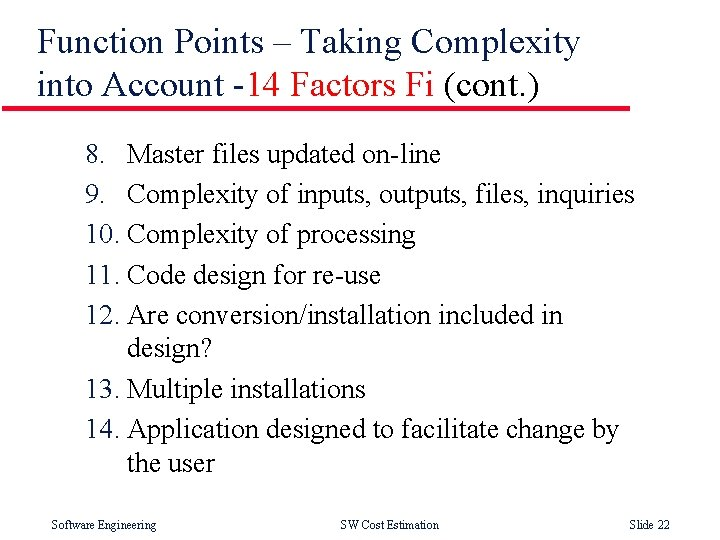Function Points – Taking Complexity into Account -14 Factors Fi (cont. ) 8. Master files updated on-line 9. Complexity of inputs, outputs, files, inquiries 10. Complexity of processing 11. Code design for re-use 12. Are conversion/installation included in design? 13. Multiple installations 14. Application designed to facilitate change by the user Software Engineering SW Cost Estimation Slide 22Function Points – Taking Complexity into Account -14 Factors Fi (cont. ) FP = UFC * [ i=14 0. 65 + 0. 01 * F i=1 i ] UFC: Unadjusted function point count 0 <= Fi <= 5 Software Engineering SW Cost Estimation Slide 23FP: Advantages & Disadvantages l Advantages Available early. . We need only a detailed specification Not restricted to code Language independent More accurate than LOC l Disadvantages Ignores quality issues of output Subjective counting. . depend on the estimator Hard to automate. . Automatic function-point counting is impossible Software Engineering SW Cost Estimation Slide 24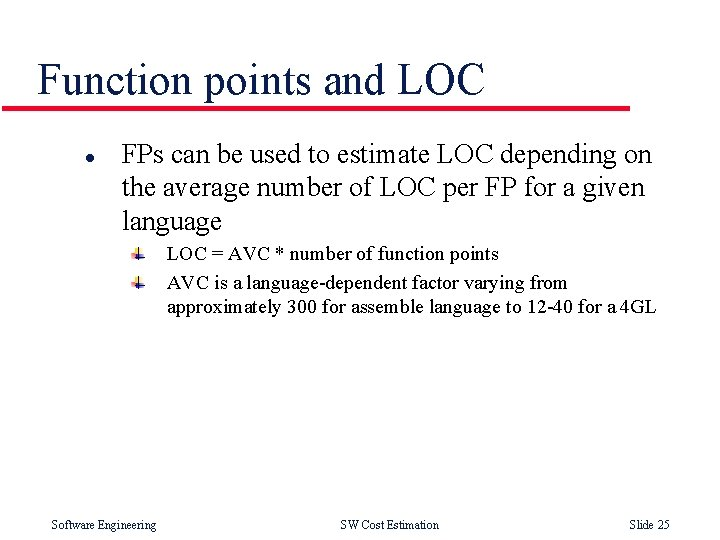Function points and LOC l FPs can be used to estimate LOC depending on the average number of LOC per FP for a given language LOC = AVC * number of function points AVC is a language-dependent factor varying from approximately 300 for assemble language to 12 -40 for a 4 GL Software Engineering SW Cost Estimation Slide 25Relation Between FP & LOC Programming Language LOC/FP (average) Assembly language 320 C 128 COBOL 106 FORTRAN 106 Pascal 90 C++ 64 Ada 53 Visual Basic 32 Smalltalk 22 Power Builder (code generator) 16 SQL 12 Software Engineering SW Cost Estimation Slide 26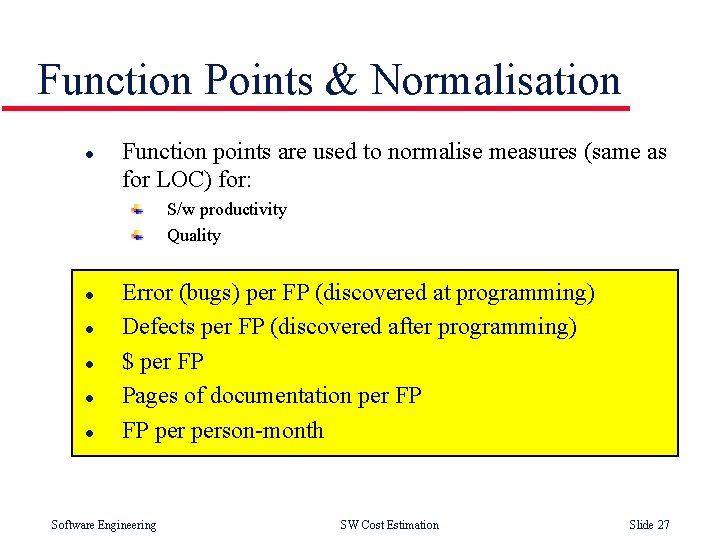Function Points & Normalisation l Function points are used to normalise measures (same as for LOC) for: S/w productivity Quality l l l Error (bugs) per FP (discovered at programming) Defects per FP (discovered after programming) \$ per FP Pages of documentation per FP FP person-month Software Engineering SW Cost Estimation Slide 27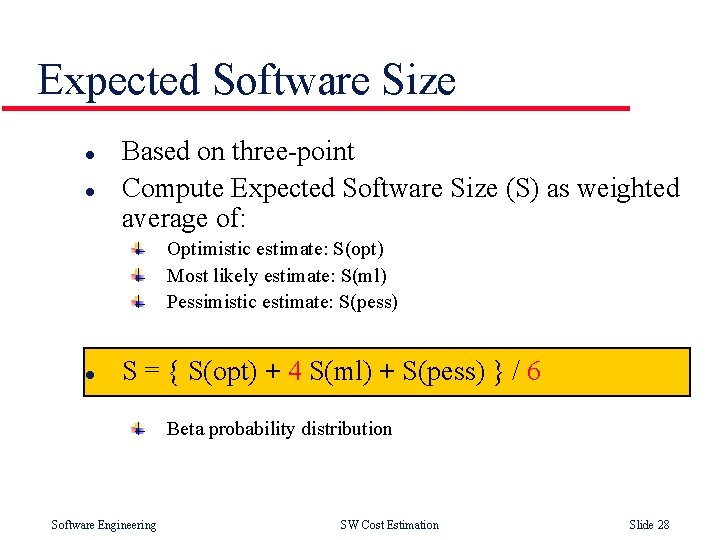Expected Software Size l l Based on three-point Compute Expected Software Size (S) as weighted average of: Optimistic estimate: S(opt) Most likely estimate: S(ml) Pessimistic estimate: S(pess) l S = { S(opt) + 4 S(ml) + S(pess) } / 6 Beta probability distribution Software Engineering SW Cost Estimation Slide 28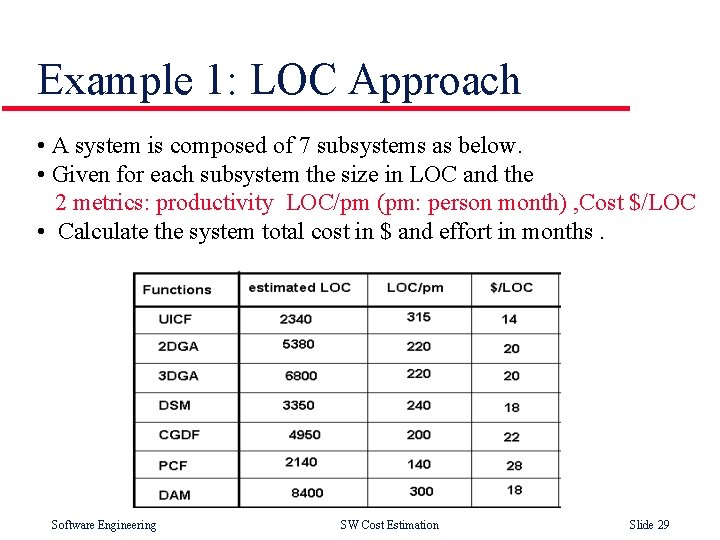Example 1: LOC Approach • A system is composed of 7 subsystems as below. • Given for each subsystem the size in LOC and the 2 metrics: productivity LOC/pm (pm: person month) , Cost \$/LOC • Calculate the system total cost in \$ and effort in months. Software Engineering SW Cost Estimation Slide 29Example 1: LOC Approach Software Engineering SW Cost Estimation Slide 30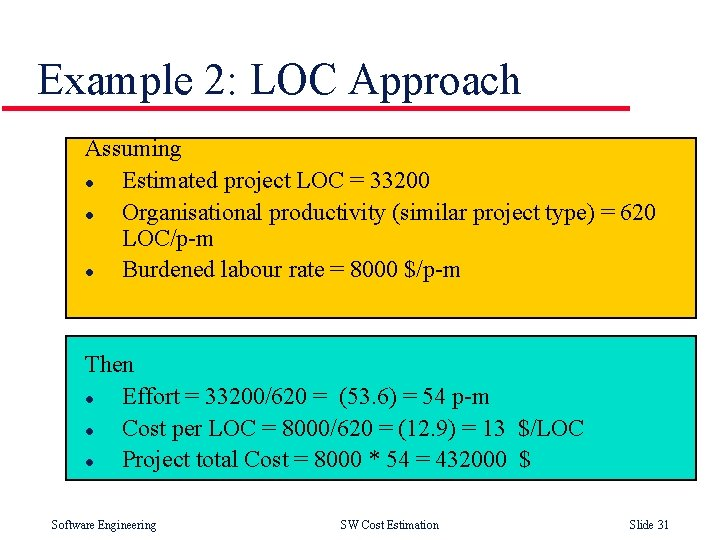Example 2: LOC Approach Assuming l Estimated project LOC = 33200 l Organisational productivity (similar project type) = 620 LOC/p-m l Burdened labour rate = 8000 \$/p-m Then l Effort = 33200/620 = (53. 6) = 54 p-m l Cost per LOC = 8000/620 = (12. 9) = 13 \$/LOC l Project total Cost = 8000 * 54 = 432000 \$ Software Engineering SW Cost Estimation Slide 31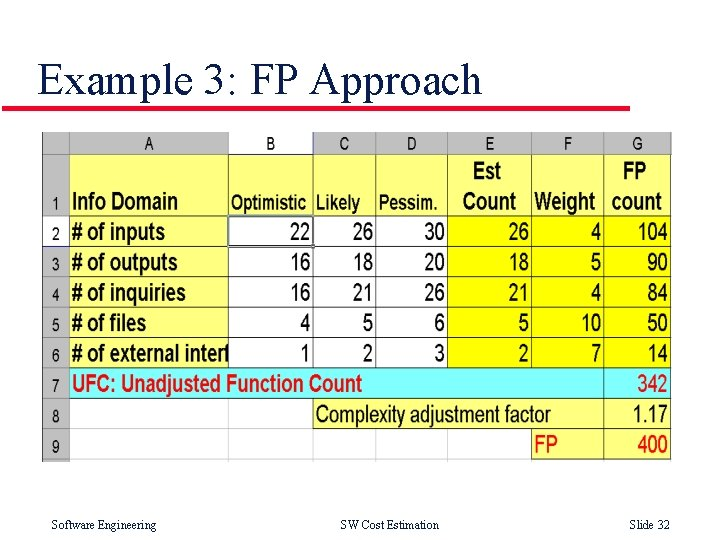Example 3: FP Approach Software Engineering SW Cost Estimation Slide 32Example 3: FP Approach (cont. ) Complexity Factor Software Engineering SW Cost Estimation Slide 33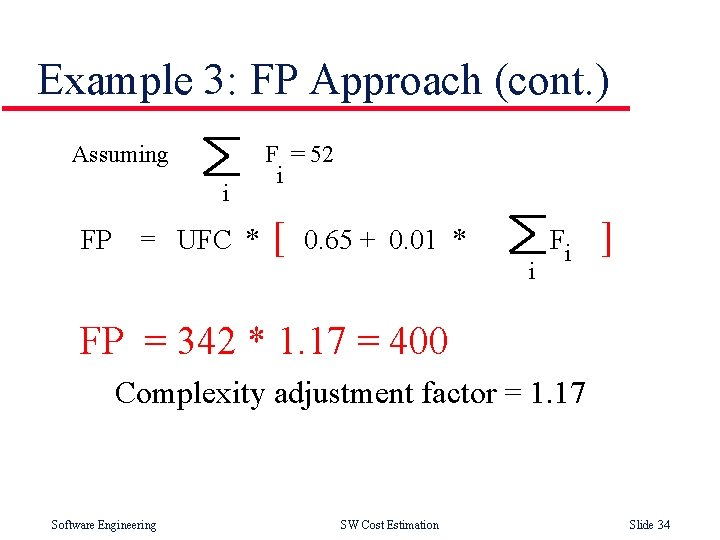Example 3: FP Approach (cont. ) Assuming i FP = UFC * F = 52 i [ 0. 65 + 0. 01 * i Fi ] FP = 342 * 1. 17 = 400 Complexity adjustment factor = 1. 17 Software Engineering SW Cost Estimation Slide 34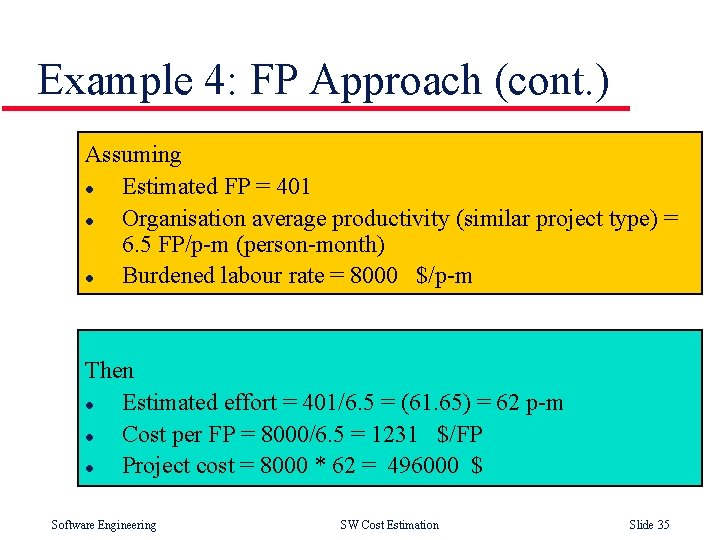Example 4: FP Approach (cont. ) Assuming l Estimated FP = 401 l Organisation average productivity (similar project type) = 6. 5 FP/p-m (person-month) l Burdened labour rate = 8000 \$/p-m Then l Estimated effort = 401/6. 5 = (61. 65) = 62 p-m l Cost per FP = 8000/6. 5 = 1231 \$/FP l Project cost = 8000 * 62 = 496000 \$ Software Engineering SW Cost Estimation Slide 35Object Points (for 4 GLs) l l l Object points are an alternative function-related measure to function points when 4 Gls or similar languages are used for development Object points are NOT the same as object classes The number of object points in a program is a weighted estimate of The number of separate screens that are displayed The number of reports that are produced by the system The number of 3 GL modules that must be developed to supplement the 4 GL code C: Software_EngCocomoSoftware Measurement Page, COCOMO II, object points. htm Software Engineering SW Cost Estimation Slide 36Object Points – Weighting Software Engineering SW Cost Estimation Slide 37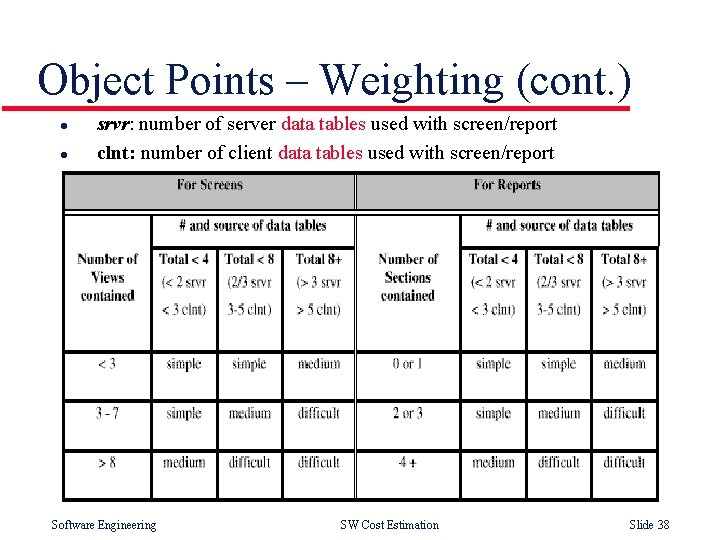Object Points – Weighting (cont. ) l l srvr: number of server data tables used with screen/report clnt: number of client data tables used with screen/report Software Engineering SW Cost Estimation Slide 38Object Point Estimation l Object points are easier to estimate from a specification than function points simply concerned with screens, reports and 3 GL modules l At an early point in the development process: Object points can be easily estimated It is very difficult to estimate the number of lines of code in a system Software Engineering SW Cost Estimation Slide 39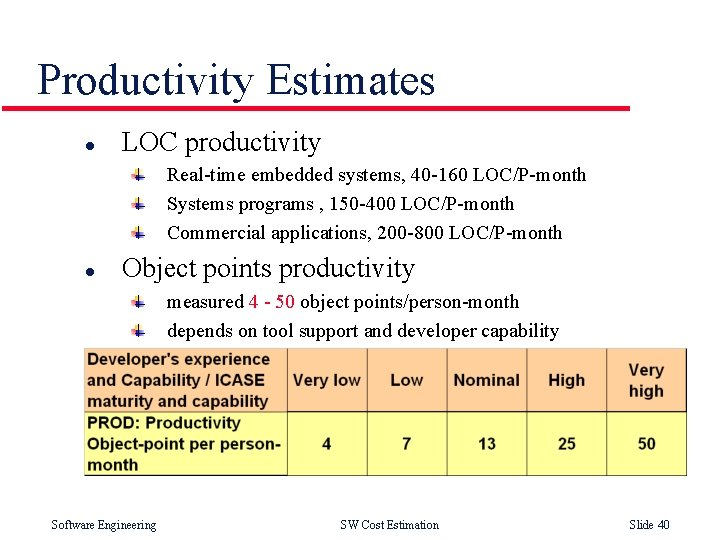Productivity Estimates l LOC productivity Real-time embedded systems, 40 -160 LOC/P-month Systems programs , 150 -400 LOC/P-month Commercial applications, 200 -800 LOC/P-month l Object points productivity measured 4 - 50 object points/person-month depends on tool support and developer capability Software Engineering SW Cost Estimation Slide 40Object Point Effort Estimation l Effort in p-m = NOP / PROD NOP = number of OP of the system Example: An application contains 840 OP (NOP=840) & Productivity is very high (= 50) Then, Effort = 840/50 = (16. 8) = 17 p-m Software Engineering SW Cost Estimation Slide 41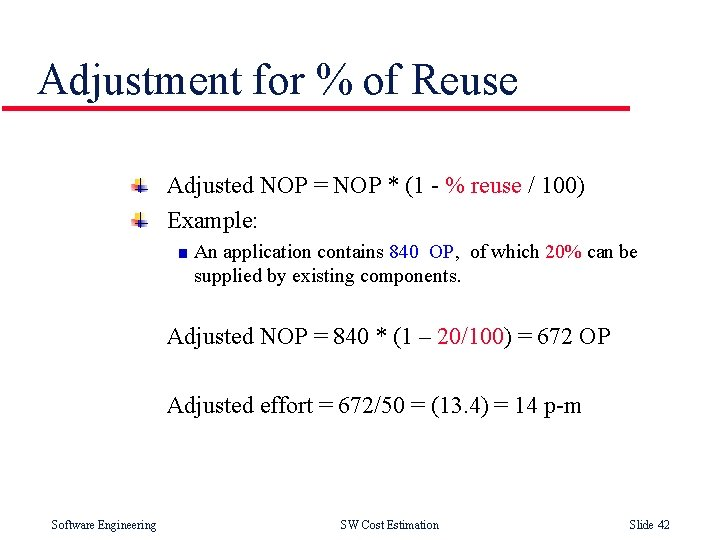Adjustment for % of Reuse Adjusted NOP = NOP * (1 - % reuse / 100) Example: An application contains 840 OP, of which 20% can be supplied by existing components. Adjusted NOP = 840 * (1 – 20/100) = 672 OP Adjusted effort = 672/50 = (13. 4) = 14 p-m Software Engineering SW Cost Estimation Slide 42Factors affecting productivity Software Engineering SW Cost Estimation Slide 43Quality and Productivity l l l All metrics based on volume/unit time are flawed because they do not take quality into account Productivity may generally be increased at the cost of quality If change is constant, then an approach based on counting lines of code (LOC) is not meaningful Software Engineering SW Cost Estimation Slide 44Estimation techniques l There is no simple way to make an accurate estimate of the effort required to develop a software system: Initial estimates may be based on inadequate information in a user requirements definition The software may run on unfamiliar computers or use new technology The people in the project may be unknown l Project cost estimates may be self-fulfilling The estimate defines the budget and the product is adjusted to meet the budget Software Engineering SW Cost Estimation Slide 45Estimation techniques l l l Algorithmic cost modelling Expert judgement Estimation by analogy Parkinson's Law Pricing to win Software Engineering SW Cost Estimation Slide 46Algorithmic code modelling l A formula – empirical relation: based on historical cost information and which is generally based on the size of the software l The formulae used in a formal model arise from the analysis of historical data. Software Engineering SW Cost Estimation Slide 47Expert Judgement l l l One or more experts in both software development and the application domain use their experience to predict software costs. Process iterates until some consensus is reached. Advantages: Relatively cheap estimation method. Can be accurate if experts have direct experience of similar systems Disadvantages: Very inaccurate if there are no experts! Software Engineering SW Cost Estimation Slide 48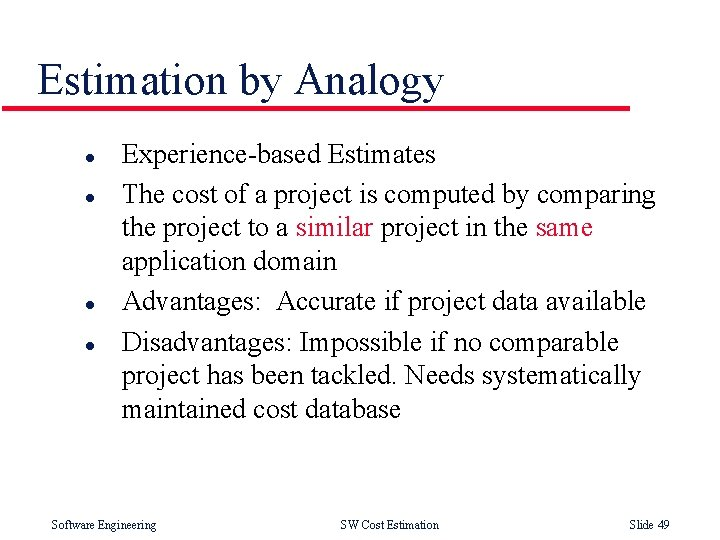Estimation by Analogy l l Experience-based Estimates The cost of a project is computed by comparing the project to a similar project in the same application domain Advantages: Accurate if project data available Disadvantages: Impossible if no comparable project has been tackled. Needs systematically maintained cost database Software Engineering SW Cost Estimation Slide 49Estimation by Analogy : Problems l However, new methods and technologies may make estimating based on experience inaccurate: Object oriented rather than function-oriented development Client-server systems rather than mainframe systems Off the shelf components Component-based software engineering CASE tools and program generators Software Engineering SW Cost Estimation Slide 50Parkinson's Law l “The project costs whatever resources are available” (Resources are defined by the software house) l l Advantages: No overspend Disadvantages: System is usually unfinished The work is contracted to fit the budget available: by reducing functionality, quality Software Engineering SW Cost Estimation Slide 51Pricing to Win l The project costs whatever the customer budget is. l Advantages: You get the contract l Disadvantages: The probability that the customer gets the system he/she wants is small. Costs do not accurately reflect the work required Software Engineering SW Cost Estimation Slide 52Pricing to Win l l This approach may seem unethical and unbusiness like However, when detailed information is lacking it may be the only appropriate strategy The project cost is agreed on the basis of an outline proposal and the development is constrained by that cost A detailed specification may be negotiated or an evolutionary approach used for system development Software Engineering SW Cost Estimation Slide 53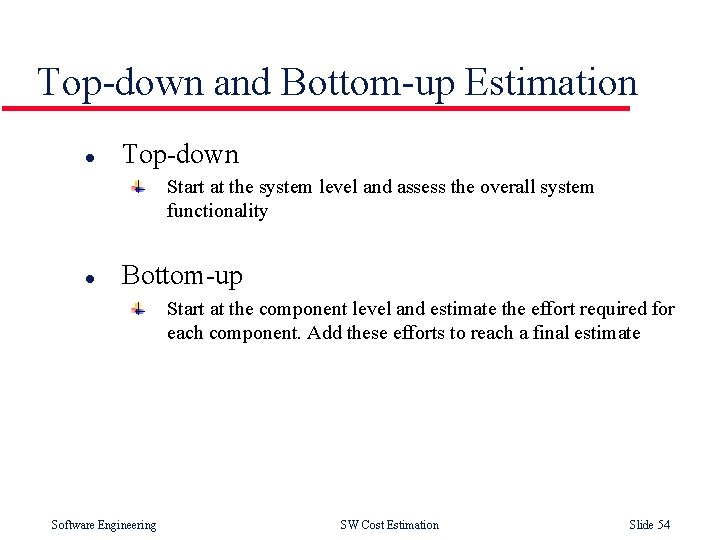Top-down and Bottom-up Estimation l Top-down Start at the system level and assess the overall system functionality l Bottom-up Start at the component level and estimate the effort required for each component. Add these efforts to reach a final estimate Software Engineering SW Cost Estimation Slide 54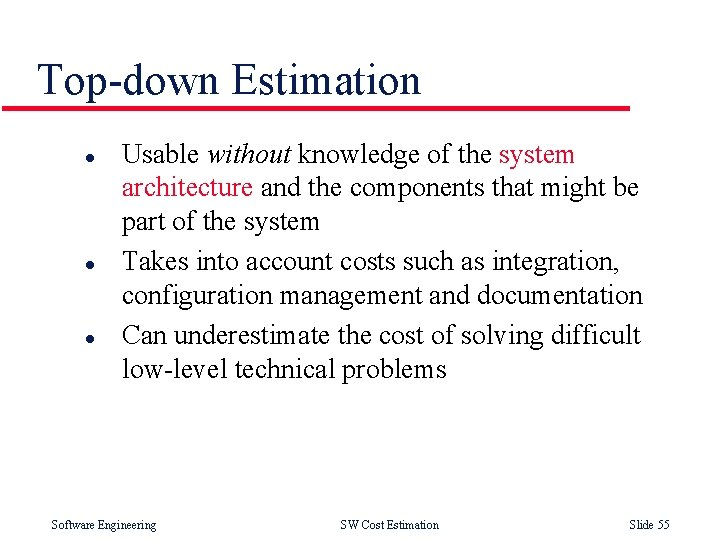Top-down Estimation l l l Usable without knowledge of the system architecture and the components that might be part of the system Takes into account costs such as integration, configuration management and documentation Can underestimate the cost of solving difficult low-level technical problems Software Engineering SW Cost Estimation Slide 55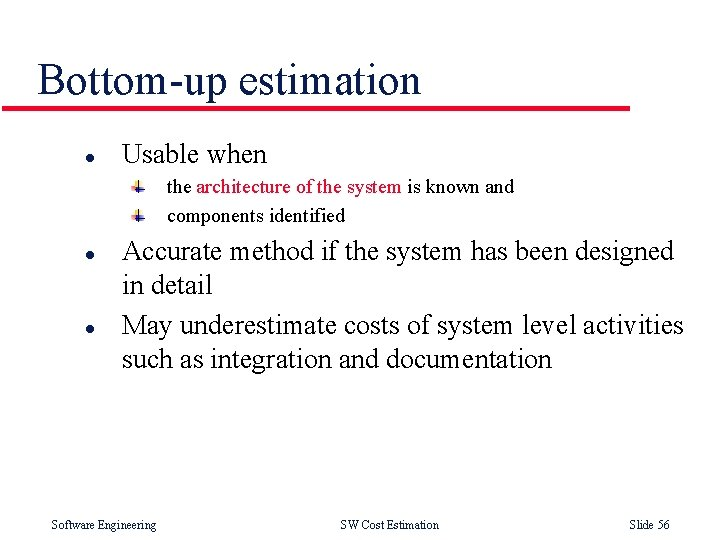Bottom-up estimation l Usable when the architecture of the system is known and components identified l l Accurate method if the system has been designed in detail May underestimate costs of system level activities such as integration and documentation Software Engineering SW Cost Estimation Slide 56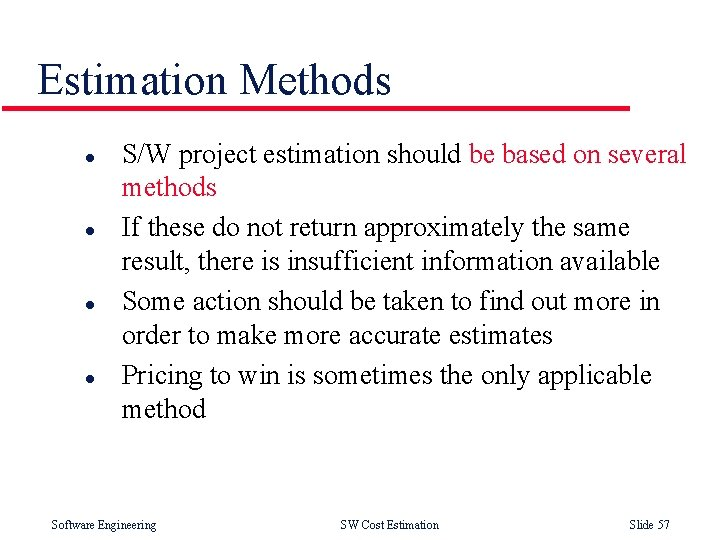Estimation Methods l l S/W project estimation should be based on several methods If these do not return approximately the same result, there is insufficient information available Some action should be taken to find out more in order to make more accurate estimates Pricing to win is sometimes the only applicable method Software Engineering SW Cost Estimation Slide 57Algorithmic Cost Modelling l l Most of the work in the cost estimation field has focused on algorithmic cost modelling. Costs are analysed using mathematical formulas linking costs or inputs with METRICS to produce an estimated output. The formula is based on the analysis of historical data. The accuracy of the model can be improved by calibrating the model to your specific development environment, (which basically involves adjusting the weighting parameters of the metrics). Software Engineering SW Cost Estimation Slide 58Building Metrics from measurements Project 1 Project 2 . . Mea sure men Analysis of historical data ts Measurements Historical Data METRICS s nt me ure s a e M Project n . . Software Engineering SW Cost Estimation Slide 59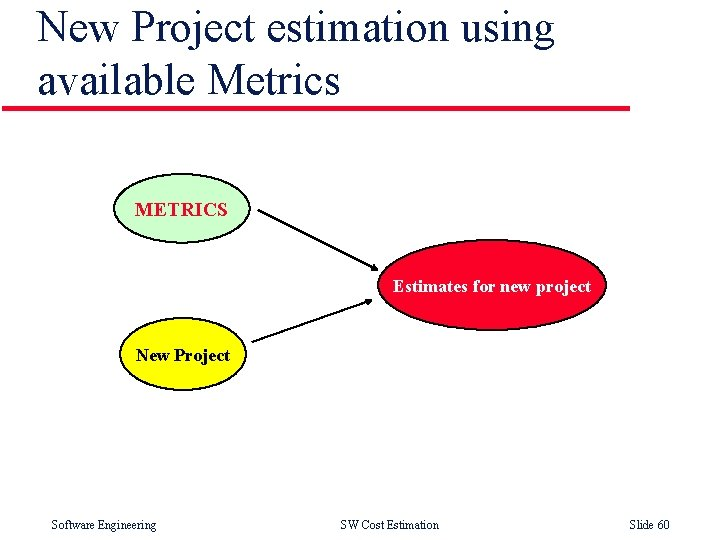New Project estimation using available Metrics METRICS Estimates for new project New Project Software Engineering SW Cost Estimation Slide 60Empirical Estimation Models Algorithmic Cost Modelling effort = tuning coefficient * size usually derived as person-months of effort required exponent usually LOC but may also be function point empirically derived either an organisation-dependent constant or a number derived based on complexity of project Software Engineering SW Cost Estimation Slide 61Algorithmic Cost Modelling Effort = A ´ Size. B ´ M A is an organisation-dependent constant B reflects the nonlinearity (disproportionate) effort for large projects M is a multiplier reflecting product, process and people attributes l l Most commonly used product attribute for cost estimation is code size (LOC) Most models are basically similar but with different values for A, B and M Software Engineering SW Cost Estimation Slide 62Estimation Accuracy l l The size of a software system can only be known accurately when it is finished Several factors influence the final size Use of COTS and components Programming language Distribution of system l As the development process progresses then the size estimate becomes more accurate Software Engineering SW Cost Estimation Slide 63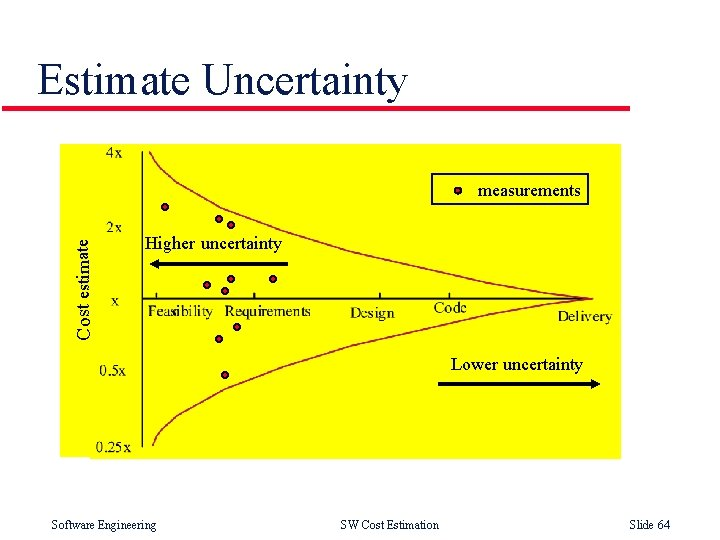Estimate Uncertainty Cost estimate measurements Higher uncertainty Lower uncertainty Software Engineering SW Cost Estimation Slide 64The COCOMO Cost model Constructive Cost Model l l An empirical model based on project experience COCOMO'81 is derived from the analysis of 63 software projects in 1981. Well-documented, ‘independent’ model which is not tied to a specific software vendor COCOMO II (2000) takes into account different approaches to software development, reuse, etc. Software Engineering SW Cost Estimation Slide 65COCOMO 81 Software Engineering SW Cost Estimation Slide 66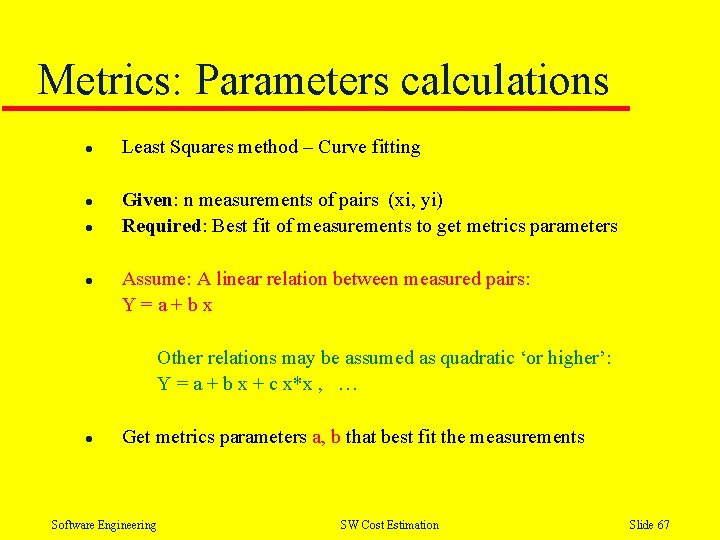Metrics: Parameters calculations l l Least Squares method – Curve fitting Given: n measurements of pairs (xi, yi) Required: Best fit of measurements to get metrics parameters Assume: A linear relation between measured pairs: Y=a+bx Other relations may be assumed as quadratic ‘or higher’: Y = a + b x + c x*x , … l Get metrics parameters a, b that best fit the measurements Software Engineering SW Cost Estimation Slide 67How to get parameters a, b Fitting Line Yi = a + b*xi Fitted Yi Measured yi Fitting Error ei = Yi - yi Measured Pair (xi, yi) Measured xi Software Engineering SW Cost Estimation Slide 68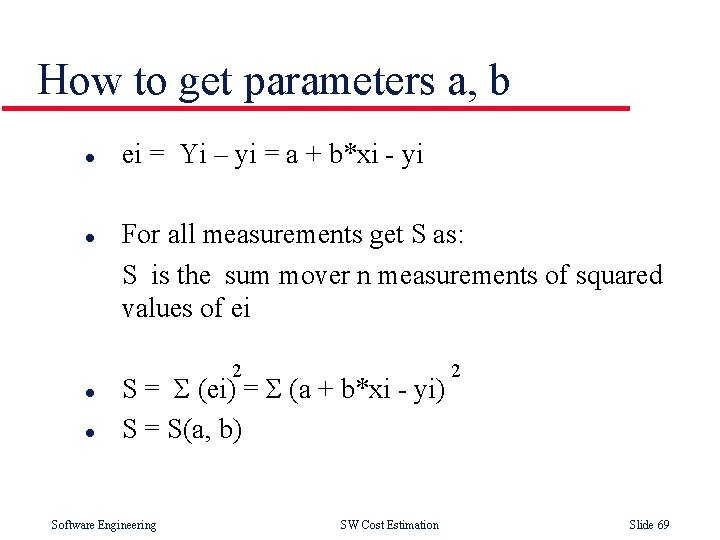How to get parameters a, b l l ei = Yi – yi = a + b*xi - yi For all measurements get S as: S is the sum mover n measurements of squared values of ei 2 l l S = Σ (ei) = Σ (a + b*xi - yi) S = S(a, b) Software Engineering SW Cost Estimation 2 Slide 69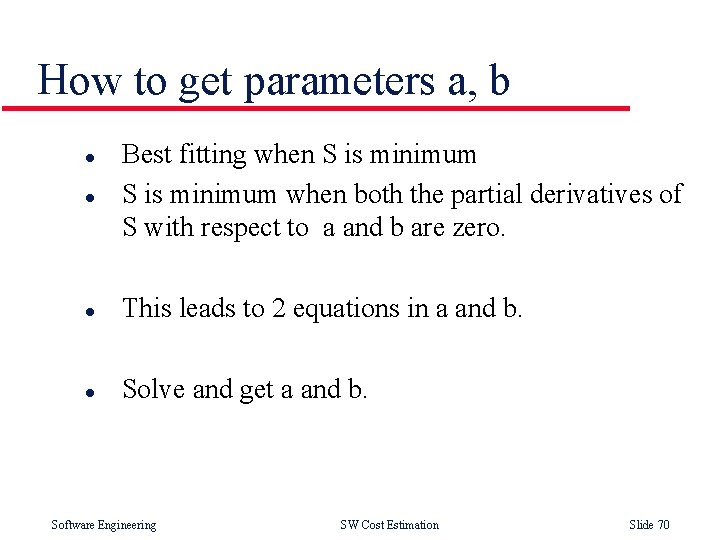How to get parameters a, b l l Best fitting when S is minimum when both the partial derivatives of S with respect to a and b are zero. l This leads to 2 equations in a and b. l Solve and get a and b. Software Engineering SW Cost Estimation Slide 70COCOMO II l COCOMO II is a 3 -level model that allows increasingly detailed estimates to be prepared as development progresses Early prototyping level Estimates based on object points and a simple formula is used for effort estimation Early design level Estimates based on function points that are then translated to LOC Includes 7 cost drivers Post-architecture level Estimates based on lines of source code or function point Includes 17 cost drivers l Five scale factors replace COCOMO 81 ratings (organic, semi -detached, and embedded) Software Engineering SW Cost Estimation Slide 71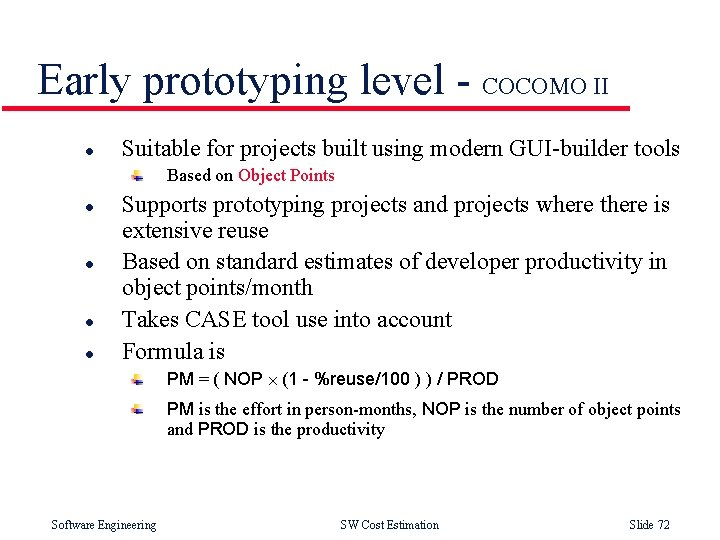Early prototyping level - COCOMO II l Suitable for projects built using modern GUI-builder tools Based on Object Points l l Supports prototyping projects and projects where there is extensive reuse Based on standard estimates of developer productivity in object points/month Takes CASE tool use into account Formula is PM = ( NOP ´ (1 - %reuse/100 ) ) / PROD PM is the effort in person-months, NOP is the number of object points and PROD is the productivity Software Engineering SW Cost Estimation Slide 72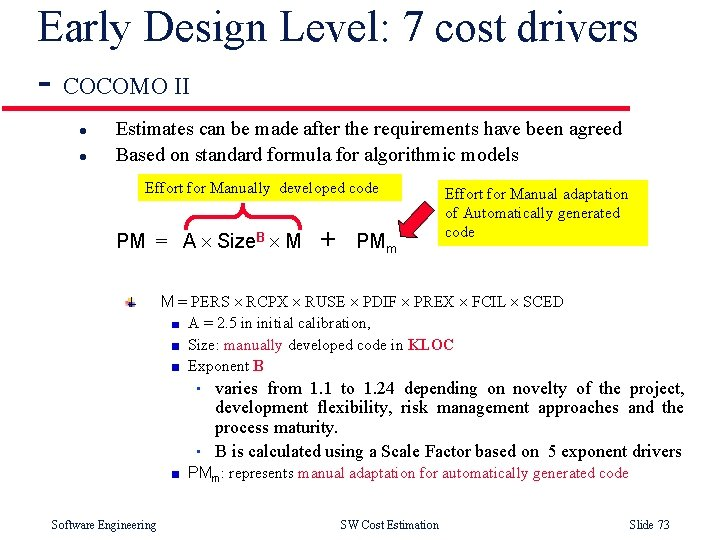Early Design Level: 7 cost drivers - COCOMO II l l Estimates can be made after the requirements have been agreed Based on standard formula for algorithmic models Effort for Manually developed code PM = A ´ Size. B ´ M + PMm Effort for Manual adaptation of Automatically generated code M = PERS ´ RCPX ´ RUSE ´ PDIF ´ PREX ´ FCIL ´ SCED A = 2. 5 in initial calibration, Size: manually developed code in KLOC Exponent B • • varies from 1. 1 to 1. 24 depending on novelty of the project, development flexibility, risk management approaches and the process maturity. B is calculated using a Scale Factor based on 5 exponent drivers PMm: represents manual adaptation for automatically generated code Software Engineering SW Cost Estimation Slide 73PMm : Manual Adaptation for Automatically Generated Code. . PMm = (ASLOC ´ (AT/100)) / ATPROD l l Used when big % of code is generated automatically ASLOC : Size of adapted components ATPROD: Productivity of the engineer integrating the adapted code (app. 2400 source statements per month) AT: % of adapted code (that is automatically generated) Software Engineering SW Cost Estimation Slide 74COCOMO II Early Design Stage Effort Multipliers: 7 cost drivers l Multipliers reflect the capability of the developers, the non-functional requirements, the familiarity with the development platform, etc. RCPX - product reliability and complexity RUSE - the reuse required PDIF - platform difficulty PREX - personnel experience PERS - personnel capability SCED - required schedule FCIL - the team support facilities Software Engineering SW Cost Estimation Slide 75The Exponent B Scale Factor(SF) - COCOMO II l l Exponent B for effort calculation B = 1. 01 + 0. 01 x sum [SF (i)] , i=1, …, 5 SF = Scale Factor l Each SF is rated on 6 -point scale (ranging from 0 to 5) : very low (5), low ( 4), nominal (3), high (2), very high (1), extra high (0) l 5 Scale Factor (exponent drivers) Precedenteness Development flexibility Architecture/risk resolution Team cohesion Process maturity Ex: 20 KLOC ^ 1. 26 / 20 KLOC ^ 1. 01 = 43. 58/20. 6 = 2. 11 Software Engineering SW Cost Estimation Slide 76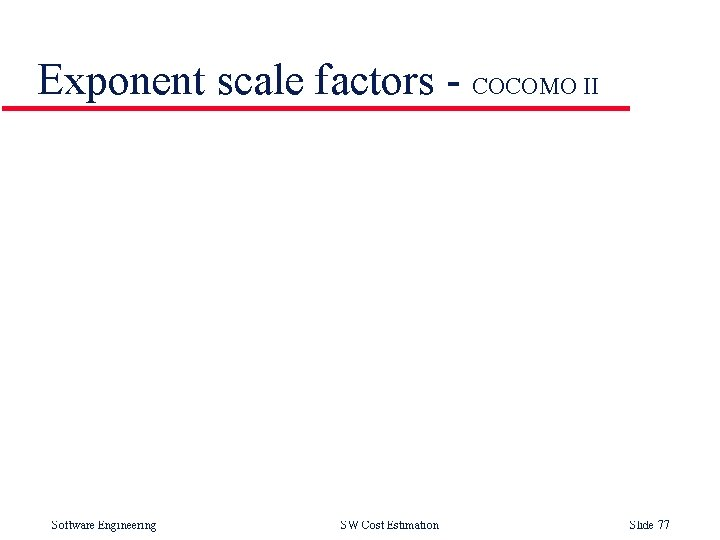Exponent scale factors - COCOMO II Software Engineering SW Cost Estimation Slide 77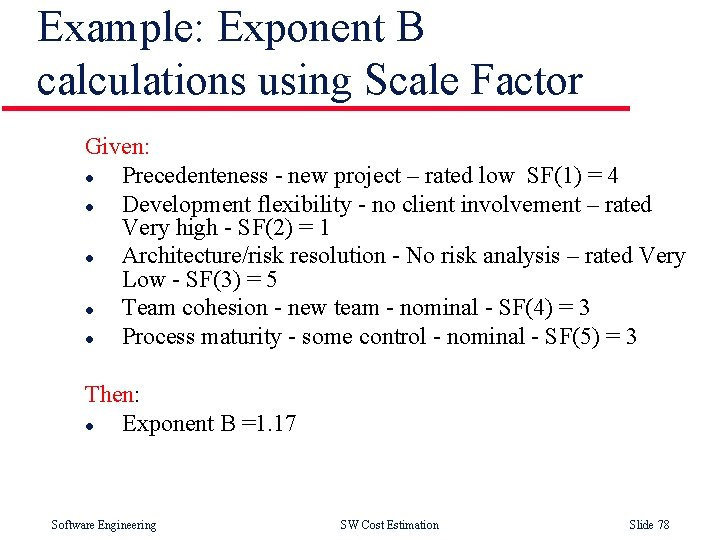Example: Exponent B calculations using Scale Factor Given: l Precedenteness - new project – rated low SF(1) = 4 l Development flexibility - no client involvement – rated Very high - SF(2) = 1 l Architecture/risk resolution - No risk analysis – rated Very Low - SF(3) = 5 l Team cohesion - new team - nominal - SF(4) = 3 l Process maturity - some control - nominal - SF(5) = 3 Then: l Exponent B =1. 17 Software Engineering SW Cost Estimation Slide 78Post-architecture stage - COCOMO II l l Uses same formula as early design estimates Estimate of size is adjusted to take into account Requirements volatility: Rework required to support change Extent of possible reuse: Reuse is non-linear and has associated costs so this is not a simple reduction in LOC ESLOC = ASLOC ´ (AA + SU +0. 4 DM + 0. 3 CM +0. 3 IM)/100 ESLOC is equivalent number of lines of new code. ASLOC is the number of lines of reusable code which must be modified, DM is the percentage of design modified, CM is the percentage of the code that is modified , IM is the percentage of the original integration effort required for integrating the reused software. SU is a factor based on the cost of software understanding, AA is a factor which reflects the initial assessment costs of deciding if software may be reused. Software Engineering SW Cost Estimation Slide 79COCOMO II Post Architecture Effort Multipliers (17 multipliers) l Product attributes (5 multipliers) concerned with required characteristics of the software product being developed l Computer attributes (3 multipliers) constraints imposed on the software by the hardware platform l Personnel attributes (6 multipliers) multipliers that take the experience and capabilities of the people working on the project into account. l Project attributes (3 multipliers) concerned with the particular characteristics of the software development project Software Engineering SW Cost Estimation Slide 80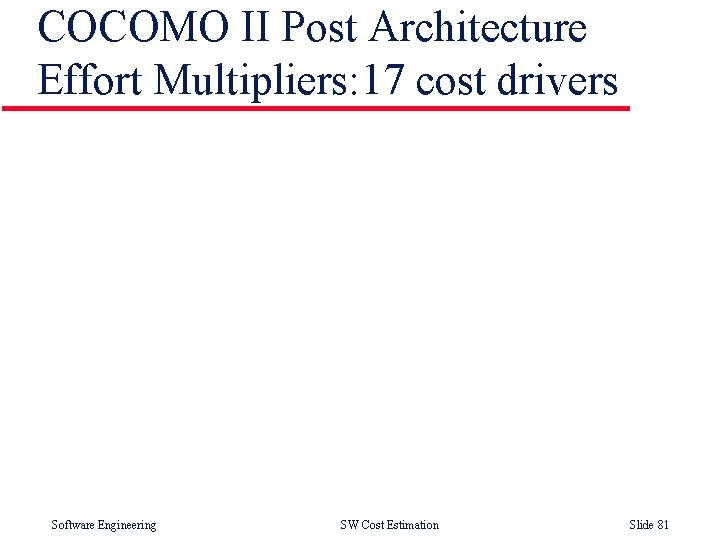COCOMO II Post Architecture Effort Multipliers: 17 cost drivers Software Engineering SW Cost Estimation Slide 81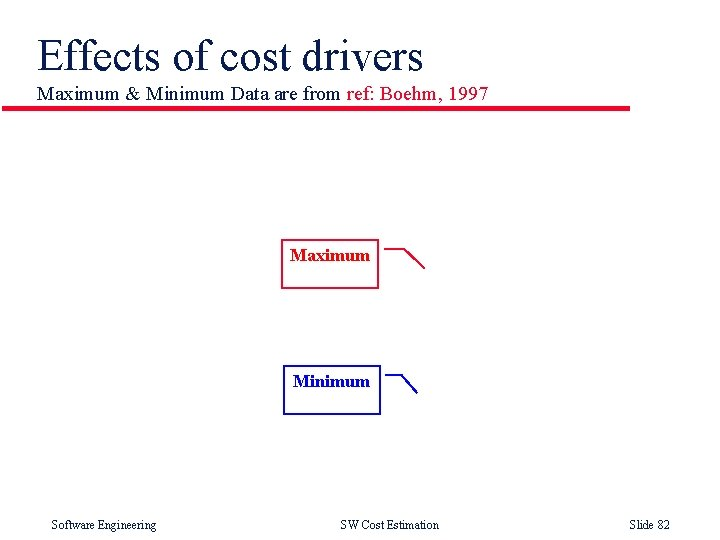Effects of cost drivers Maximum & Minimum Data are from ref: Boehm, 1997 Maximum Minimum Software Engineering SW Cost Estimation Slide 82Effects of cost drivers (M = ? ) Maximum & Minimum Data are from ref: Boehm, 1997 Software Engineering SW Cost Estimation Slide 83Project planning l l Algorithmic cost models provide a basis for project planning as they allow alternative strategies to be compared Embedded spacecraft system Must be reliable Must minimise weight (number of chips) Multipliers on reliability and computer constraints > 1 l Cost components Target hardware Development platform Effort required Software Engineering SW Cost Estimation Slide 84Management options Software Engineering SW Cost Estimation Slide 85Management options costs Software Engineering SW Cost Estimation Slide 86Option choice l Option D (use more experienced staff) appears to be the best alternative However, it has a high associated risk as experienced staff may be difficult to find l l Option C (upgrade memory) has a lower cost saving but very low risk Overall, the model reveals the importance of staff experience in software development Software Engineering SW Cost Estimation Slide 87Project duration and staffing - COCOMO II l l As well as effort estimation, managers must estimate the calendar time required to complete a project and when staff will be required Calendar time can be estimated using a COCOMO II formula TDEV = 3 ´ (PM)(0. 33+0. 2*(B-1. 01)) PM is the effort computation and B is the exponent computed as discussed above (B is 1 for the early prototyping model). This computation predicts the nominal schedule for the project l The time required is independent of the number of people working on the project Software Engineering SW Cost Estimation Slide 88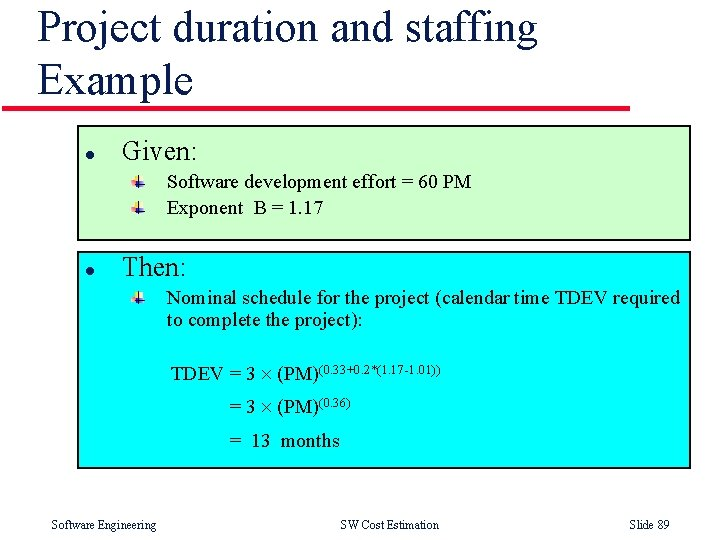Project duration and staffing Example l Given: Software development effort = 60 PM Exponent B = 1. 17 l Then: Nominal schedule for the project (calendar time TDEV required to complete the project): TDEV = 3 ´ (PM)(0. 33+0. 2*(1. 17 -1. 01)) = 3 ´ (PM)(0. 36) = 13 months Software Engineering SW Cost Estimation Slide 89Staffing requirements l l Staff required can’t be computed by diving the development time by the required schedule – Non linear relation ship The number of people working on a project varies depending on the phase of the project The more people who work on the project, the more total effort is usually required A very rapid build-up of people often correlates with schedule slippage Software Engineering SW Cost Estimation Slide 90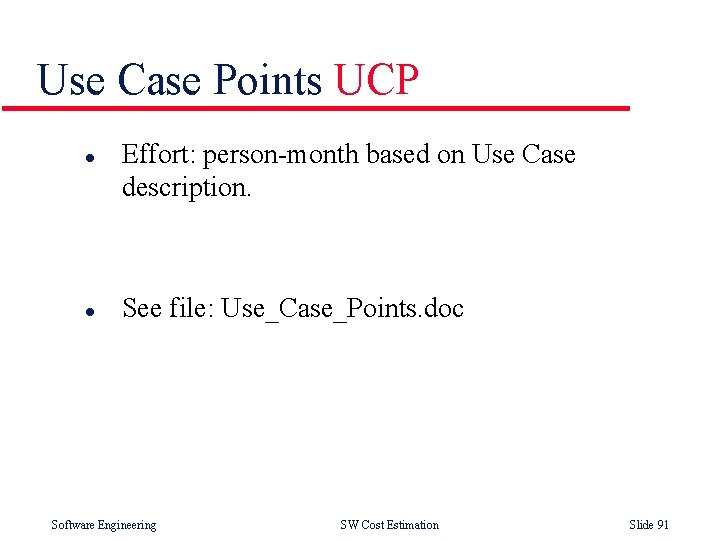Use Case Points UCP l l Effort: person-month based on Use Case description. See file: Use_Case_Points. doc Software Engineering SW Cost Estimation Slide 91